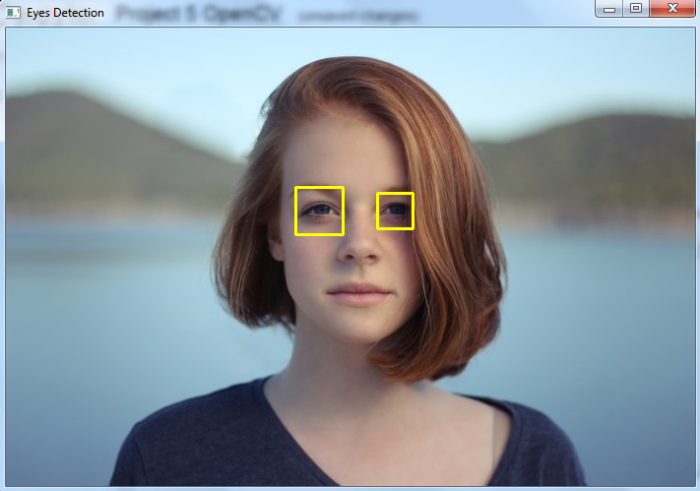# How to detect eyes in an image using OpenCV Python?

A "haar cascade classifier" is an effective machine learning based approach for object detection. To train a haar cascade classifier for eye detection, the algorithm initially needs a lot of positive images (images of eyes) and negative images (images without eyes). Then the classifier is trained from these positive and negative images. It is then used to detect eyes in other images. We can use already trained haar cascades for eye detection.

For eye detection in the input image, we need two haar cascades one for face detection and other for eye detection. We will use the following two haar cascades −

## Steps

To detect eyes in an image and draw bounding boxes around them, you can follow the steps given below −

• Import the required library. In all the following examples, the required Python library is OpenCV. Make sure you have already installed it.

• Read the input image using cv2.imread() in a grayscale. Specify the full image path.

• Detect faces in the input image using face_cascade.detectMultiScale(). It returns the coordinates of detected faces in (x,y,w,h) format.

• Define roi as image[y:y+h, x:x+w] for the detected face. Now detect eyes within the detected face area (roi). Use eye_cascade.detectMultiScale(). It also returns the coordinate of the bounding rectangle of eyes in (ex,ey,ew,eh) format.

• Draw the bounding rectangles around the detected eyes in the original image using cv2.rectangle().

• Display the image with the drawn bounding rectangles around the eyes.

Let's have a look at some examples for more clear understanding.

## Example

In this Python program, we detect eyes in the input image using a haar cascade.

# import required libraries
import cv2

# convert to grayscale of each frames
gray = cv2.cvtColor(img, cv2.COLOR_BGR2GRAY)

# detects faces in the input image
print('Number of detected faces:', len(faces))

# loop over the detected faces
for (x,y,w,h) in faces:
roi_gray = gray[y:y+h, x:x+w]
roi_color = img[y:y+h, x:x+w]

# detects eyes of within the detected face area (roi)

# draw a rectangle around eyes
for (ex,ey,ew,eh) in eyes:
cv2.rectangle(roi_color,(ex,ey),(ex+ew,ey+eh),(0,255,255),2)

# display the image with detected eyes
cv2.imshow('Eyes Detection',img)
cv2.waitKey(0)
cv2.destroyAllWindows()


Consider the following image as the Input File for this program −When you run the above Python program, it will produce the following output window −

Number of detected faces: 1


And we get the following output window showing the detected eyes in the image -The bounding boxes around the detected eyes are drawn with yellow color.